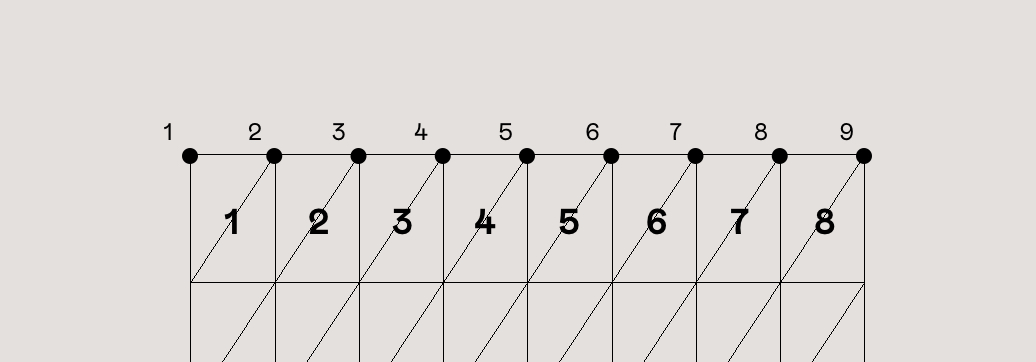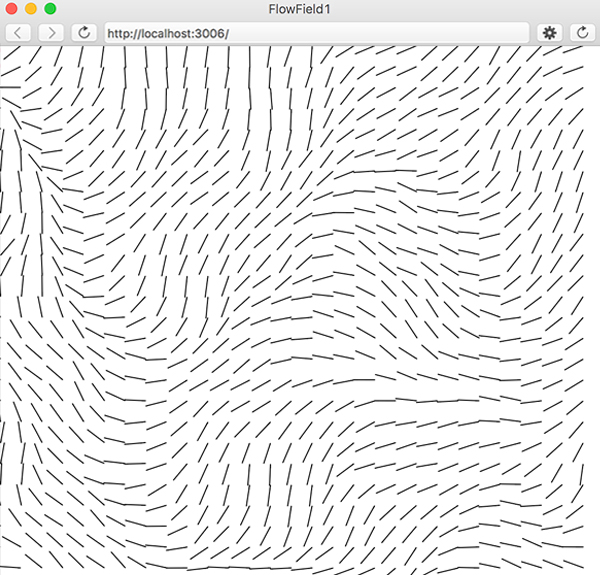# How to Create a Physics-based 3D Cloth with Cannon.js and Three.js

Following my previous experiment where I’ve showed you how to build a 3D physics-based menu, let’s now take a look at how to turn an image into a cloth-like material that gets distorted by wind using Cannon.js and Three.js.

In this tutorial, we’ll assume that you’re comfortable with Three.js and understand the basic principles of the Cannon.js library. If you aren’t, take a look at my previous tutorial about Cannon and how to create a simple world using this 3D engine.

Before we begin, take a look at the demo that shows a concrete example of a slideshow that uses the cloth effect I’m going to explain. The slideshow in the demo is based on Jesper Landberg’s Infinite draggable WebGL slider.

Preparing the DOM, the scene and the figure

I’m going to start with an example from one of my previous tutorials. I’m using DOM elements to re-create the plane in my scene. All the styles and positions are set in CSS and re-created in the canvas with JavaScript. I just cleaned some stuff I don’t use anymore (like the data-attributes) but the logic is still the same:

// index.html
<section class=”container”>
<article class=”tile”>
<figure class=”tile__figure”>
<img src=”path/to/my/image.jpg”
class=”tile__image” alt=”My image” width=”400″
height=”300″ />
</figure>
</article>
</section>

And here we go:

Creating the physics world and update existing stuff

We’ll update our Scene.js file to add the physics calculation and pass the physics World as an argument to the Figure object:

// Scene.js’s constructor
this.world = new C.World();
this.world.gravity.set(0, -1000, 0);

For this example, I’m using a large number for gravity because I’m working with big sized objects.

// Scene.js’s constructor
this.figure = new Figure(this.scene, this.world);

// Scene.js’s update method
this.world.step(1 / 60);

// We’ll see this below!
this.figure.update()

Let’s do some sewing

In the last tutorial on Cannon, I talked about rigid bodies. As its name suggests, you give an entire object a shape that will never be distorted. In this example, I will not use rigid bodies but soft bodies. I’ll create a new body per vertex, give it a mass and connect them to recreate the full mesh. After that, like with the rigid bodies, I copy each Three vertices’ position with Cannon’s body position and voilà!

Let’s start by updating the subdivision segments of the mesh with a local variable “size”:

const size = 8;

export default class Figure {
constructor(scene, world) {
this.world = world

//…

// Createmesh method
this.geometry = new THREE.PlaneBufferGeometry(1, 1, size, size);

Then, we add a new method in our Figure Class called “CreateStitches()” that we’ll call it just after the createMesh() method. The order is important because we’ll use each vertex coordinate to set the base position of our bodies.

Creating the soft body

Because I’m using a BufferGeometry rather than Geometry, I have to loop through the position attributes array based on the count value. It limits the number of iterations through the whole array and improves performances. Three.js provides methods that return the correct value based on the index.

createStitches() {
// We don’t want a sphere nor a cube for each point of our cloth. Cannon provides the Particle() object, a shape with … no shape at all!
const particleShape = new C.Particle();

const { position } = this.geometry.attributes;
const { x: width, y: height } = this.sizes;

this.stitches = [];

for (let i = 0; i < position.count; i++) {

const pos = new C.Vec3(
position.getX(i) * width,
position.getY(i) * height,
position.getZ(i)
);

const stitch = new C.Body({

// We divide the mass of our body by the total number of points in our mesh. This way, an object with a lot of vertices doesn’t have a bigger mass.
mass: mass / position.count,

// Just for a smooth rendering, you can drop this line but your cloth will move almost infinitely.
linearDamping: 0.8,

position: pos,
shape: particleShape,

// TEMP, we’ll delete later
velocity: new C.Vec3(0, 0, -300)
});

this.stitches.push(stitch);
}
}

Notice that we multiply by the size of our mesh. That’s because, in the beginning, we set the size of our plane to a size of 1. So each vertex has its coordinates normalized and we have to multiply them afterwards.Updating the mesh

As we need to set our position in normalized coordinates, we have to divide by the width and height values and set it to the bufferAttribute.

// Figure.js
update() {
const { position } = this.geometry.attributes;
const { x: width, y: height } = this.sizes;

for (let i = 0; i < position.count; i++) {
position.setXYZ(
i,
this.stitches[i].position.x / width,
this.stitches[i].position.y / height,
this.stitches[i].position.z
);
}

position.needsUpdate = true;
}

And voilà! Now you should have a falling bunch of unconnected points. Let’s change that by just setting the first row of our stitches to a mass of zero.

for (let i = 0; i < position.count; i++) {
const row = Math.floor(i / (size + 1));

// …

const stitch = new C.Body({
mass: row === 0 ? 0 : mass / position.count,

// …

I guess you noticed I increased the size plus one. Let’s take a look at the wireframe of our mesh:As you can notice, when we set the number of segments with the ‘size’ variable, we have the correct number of subdivisions. But we are working on the mesh so we have one more row and column. By the way, if you inspect the count value we used above, we have 81 vertices (9*9), not 64 (8*8).

Connecting everything

Now, you should have a falling bunch of points falling down but not the first line! We have to create a DistanceConstraint from each point to their neighbour.// createStitches()
for (let i = 0; i < position.count; i++) {
const col = i % (size + 1);
const row = Math.floor(i / (size + 1));

if (col < size) this.connect(i, i + 1);
if (row < size) this.connect(i, i + size + 1);
}

// New method in Figure.js
connect(i, j) {
const c = new C.DistanceConstraint(this.stitches[i], this.stitches[j]);

}

And tadam! You now have a cloth floating within the void. Because of the velocity we set before, you can see the mesh moves but stops quickly. It’s the calm before the storm.

Let the wind blow

Now that we have a cloth, why not let a bit of wind blow? I’m going to create an array with the length of our mesh and fill it with a direction vector based on the position of my mouse multiplied by a force using simplex noise. Psst, if you have never heard of noise, I suggest reading this article.

We could imagine the noise looking like this image, except where we have angles in each cell, we’ll have a force between -1 and 1 in our case.https://lramrz.com/2016/12/flow-field-in-p5js/

After that, we’ll add the forces of each cell on their respective body and the update function will do the rest.

Let’s dive into the code!

I’m going to create a new class called Wind in which I’m passing the figure as a parameter.

// First, I’m going to set 2 local constants
const baseForce = 2000;
const off = 0.05;

export default class Wind {
constructor(figure) {
const { count } = figure.geometry.attributes.position;
this.figure = figure;

// Like the mass, I don’t want to have too much forces applied because of a large amount of vertices
this.force = baseForce / count;

// We’ll use the clock to increase the wind movement
this.clock = new Clock();

// Just a base direction
this.direction = new Vector3(0.5, 0, -1);

// My array
this.flowfield = new Array(count);

// Where all will happen!
this.update()
}
}

update() {
const time = this.clock.getElapsedTime();

const { position } = this.figure.geometry.attributes;
const size = this.figure.geometry.parameters.widthSegments;

for (let i = 0; i < position.count; i++) {
const col = i % (size + 1);
const row = Math.floor(i / (size + 1));

const force = (noise.noise3D(row * off, col * off, time) * 0.5 + 0.5) * this.force;

this.flowfield[i] = this.direction.clone().multiplyScalar(force);
}
}

The only purpose of this object is to update the array values with noise in each frame so we need to amend Scene.js with a few new things.

// Scene.js
this.wind = new Wind(this.figure.mesh);

// …

update() {
// …
this.wind.update();
this.figure.update();
// …
}

And before continuing, I’ll add a new method in my update method after the figure.update():

this.figure.applyWind(this.wind);

Let’s write this new method in Figure.js:

// Figure.js constructor
// To help performance, I will avoid creating a new instance of vector each frame so I’m setting a single vector I’m going to reuse.
this.bufferV = new C.Vec3();

// New method
applyWind(wind) {
const { position } = this.geometry.attributes;

for (let i = 0; i < position.count; i++) {
const stitch = this.stitches[i];

const windNoise = wind.flowfield[i];
const tempPosPhysic = this.bufferV.set(
windNoise.x,
windNoise.y,
windNoise.z
);

stitch.applyForce(tempPosPhysic, C.Vec3.ZERO);
}
}

Congratulation, you have created wind, Mother Nature would be proud! But the wind blows in the same direction. Let’s change that in Wind.js by updating our direction with the mouse position.

onMouseMove({ clientX: x, clientY: y }) {
const { innerWidth: W, innerHeight: H } = window;

gsap.to(this.direction, {
duration: 0.8,
x: x / W – 0.5,
y: -(y / H) + 0.5
});
}

Conclusion

I hope you enjoyed this tutorial and that it gave you some ideas on how to bring a new dimension to your interaction effects. Don’t forget to take a look at the demo, it’s a more concrete case of a slideshow where you can see this effect in action.

Don’t hesitate to let me know if there’s anything not clear, feel free to contact me on Twitter @aqro.

Cheers!

How to Create a Physics-based 3D Cloth with Cannon.js and Three.js was written by Arno Di Nunzio and published on Codrops.0 replies